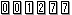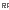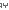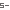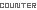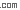LastUpdate 3/10/2022

Japanese (日本語版)This software is a simuration software that uses the Monte Carlo method to obtain an approximate value of pi. The Monte Carlo method is a general term for methods that perform simulations and numerial calculations using random numbers. 　There　are softfares created in Java for simulating on a personal computer and apps created in Android for simulaing on a smartphone. (For the Android version , see "Interesting Android App" in Menu 1.) 　As an example of finding an approximate value of pi using the Monte Carlo method , it is common to "Scatter sesame seeds to find an approximate value of pi." (Draw a square and a circle inscribed in it , and randomly scatter sesame seeds from above. Calculate the approximate value of pi from the ratio of the number of sesame seeds in a square to the number of sesame seeds in a circle.)Draw several parallel lines with a width equal to the diameter of the coin to be thrown. In addition , draw several parallel lines that are the same width as these parallel lines and are orthogonal to each other (Hereafter , it is called a grid line). Randomly throw coins onto this grid. From the ratio of the total number of coins thrown to the number of coins that overlap the grid points , calculate the approximate value of pi. (The intersection of parallel lines on a grid line is called a grid point.) 　The following formula is caluculated to obtain an approximate value of pi. 　π = 4 × (The number of coins that overlap the grid points) ÷ (Total number of coins thrown) 　The number of coins to be thrown is , 10 , 100 , 1000 , 10000 , and 50000.OS: Confirmed to work on Windows Vista / 7 / 8 / 10 You must have the JDK installed according to the "Setup Method" below.To use the simulation software "Throw coins to find an approximate value of pi !" , you need to set it up on your hard disk. 　Even if you say setup , all you have to do is to decompress the downloaded compressed file and copy the created folder to the hard disk of the computer you want to use. 　Java application can be operated only in the enviroment where JRE (Java SE excution environment) is installed. Download and install the Java Development Kit (JDK) from Oracle website. (free)Double-click batch file "〜.bat" in the folder created on the drive you set up.Double-click batch file "〜.bat" to start this software. 　Select the number of coins to throw from the  button to the  button and click. 　The number of coins that overlap the grid points and the approximate value of pi at that time are displayed. 　Click the [Init] button to clear the screen. 　Click the [Exit] button to exit this software.Simulation software 『Throw coins to find an approximate value of pi！（Java）』 executable file

 Simulation software 『Scatter sesame seeds to find an approximate value of pi！（Java）』 executable file

 Simulation software 『Scatter needles to find an approximate value of pi！（Java）』 executable file